## Hyperboloids

 TWO-SHEETED HYPERBOLOIDS
 two-sheeted elliptical hyperboloid $$-\frac{x^2}{a^2}-\frac{y^2}{b^2}+\frac{z^2}{c^2}=1$$ Real plane intersections are: the ellipses, the circles, the parabolas and the hyperbolas. hyperboloid of revolution, two-sheeted circular hyperboloid $$-\frac{x^2}{a^2}-\frac{y^2}{a^2}+\frac{z^2}{c^2}=1$$ It is obtained by rotating a hyperbola about its transverse axis. Real plane intersections are: the ellipses,the circles, the parabolas and the hyperbolas.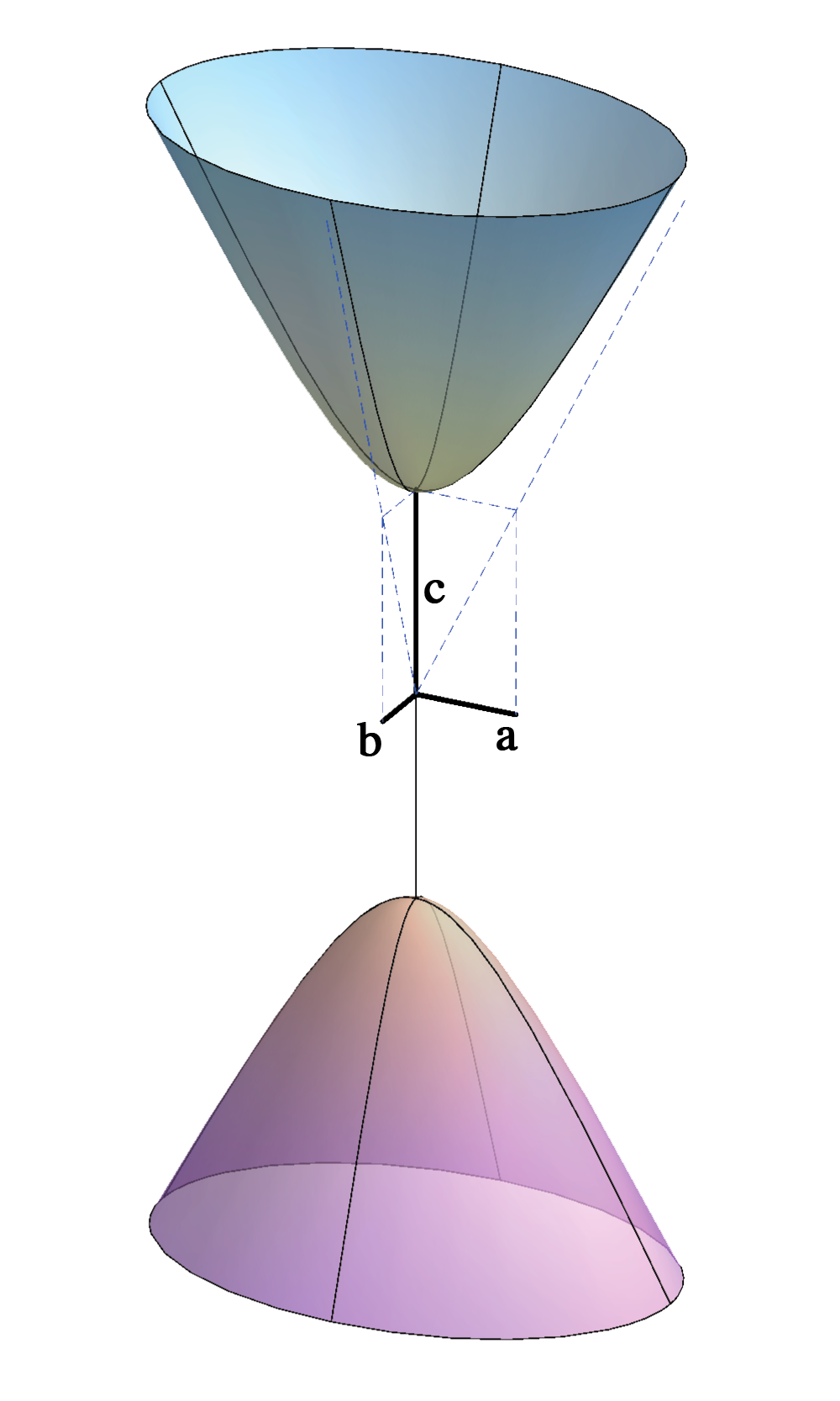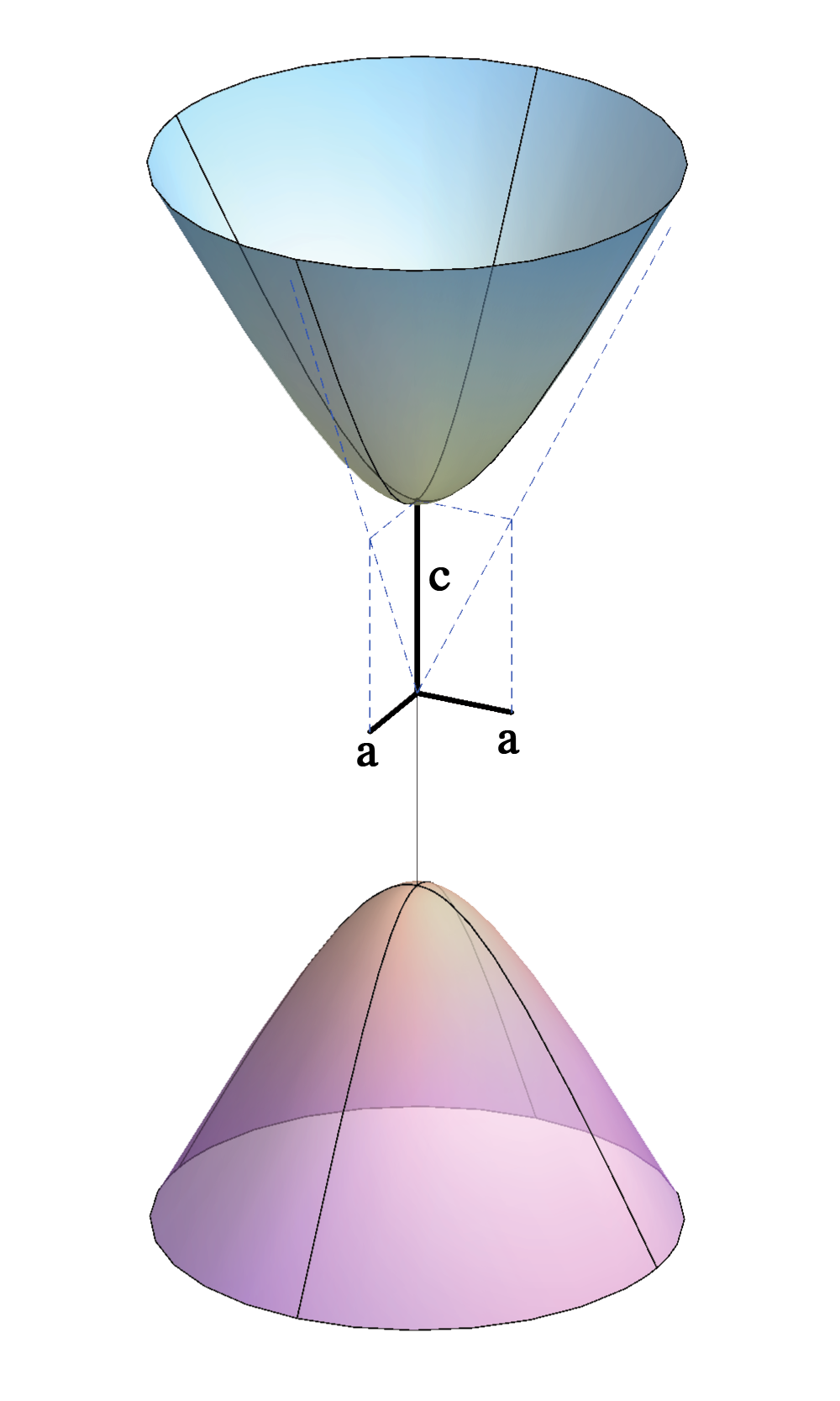right-click on the image $$\rightarrow$$ PLAY
 ONE-SHEETED HYPERBOLOID one-sheeted elliptical hyperboloid $$\frac{x^2}{a^2}+\frac{y^2}{b^2}-\frac{z^2}{c^2}=1$$ A ruled surface with two systems of rulings - there are two lines of the surface through each point of this surface. Real plane intersections are: the ellipses, the circles, the parabolas, the hyperbolas and degenerated conics - pairs of two real lines (intersections with the tangent planes).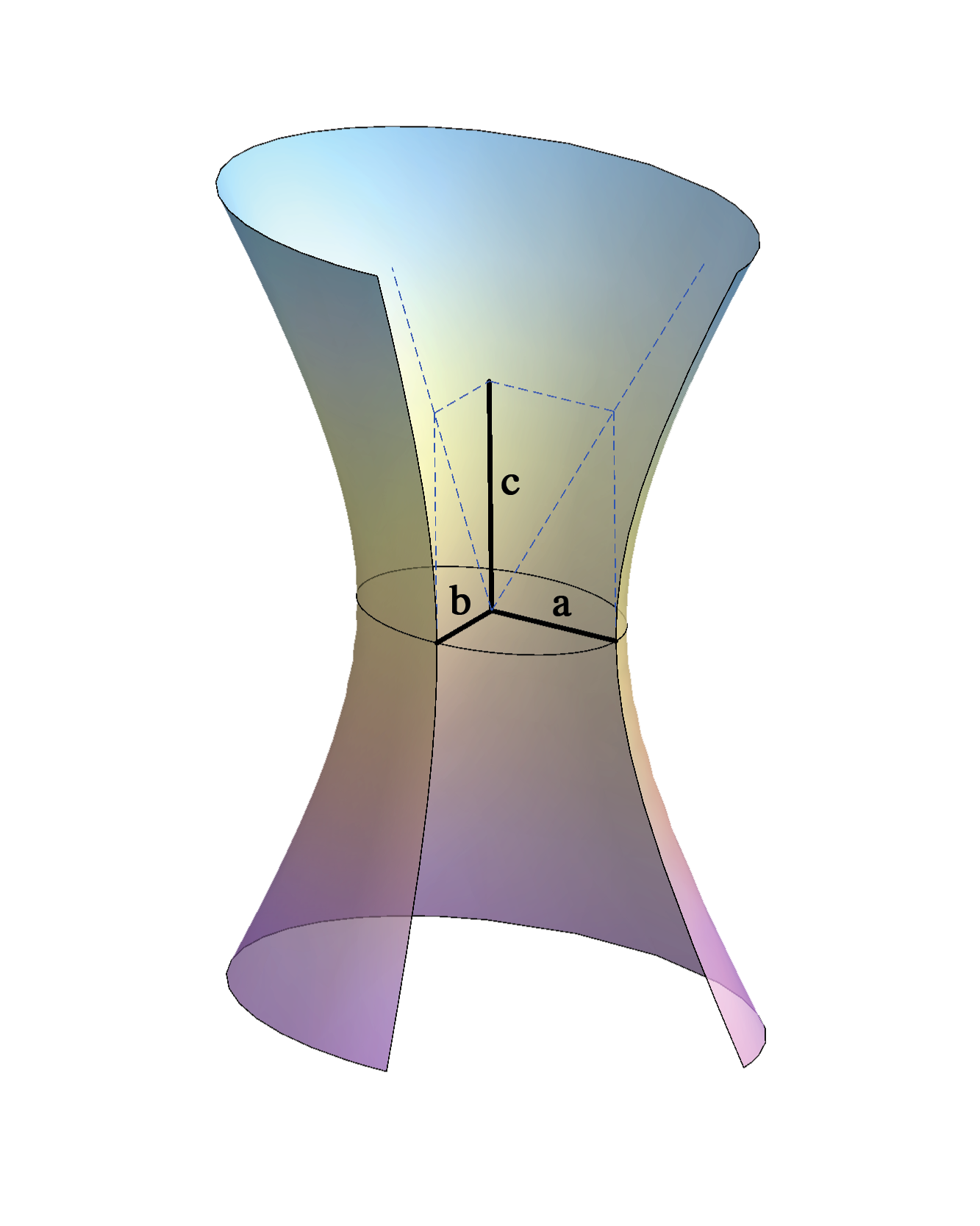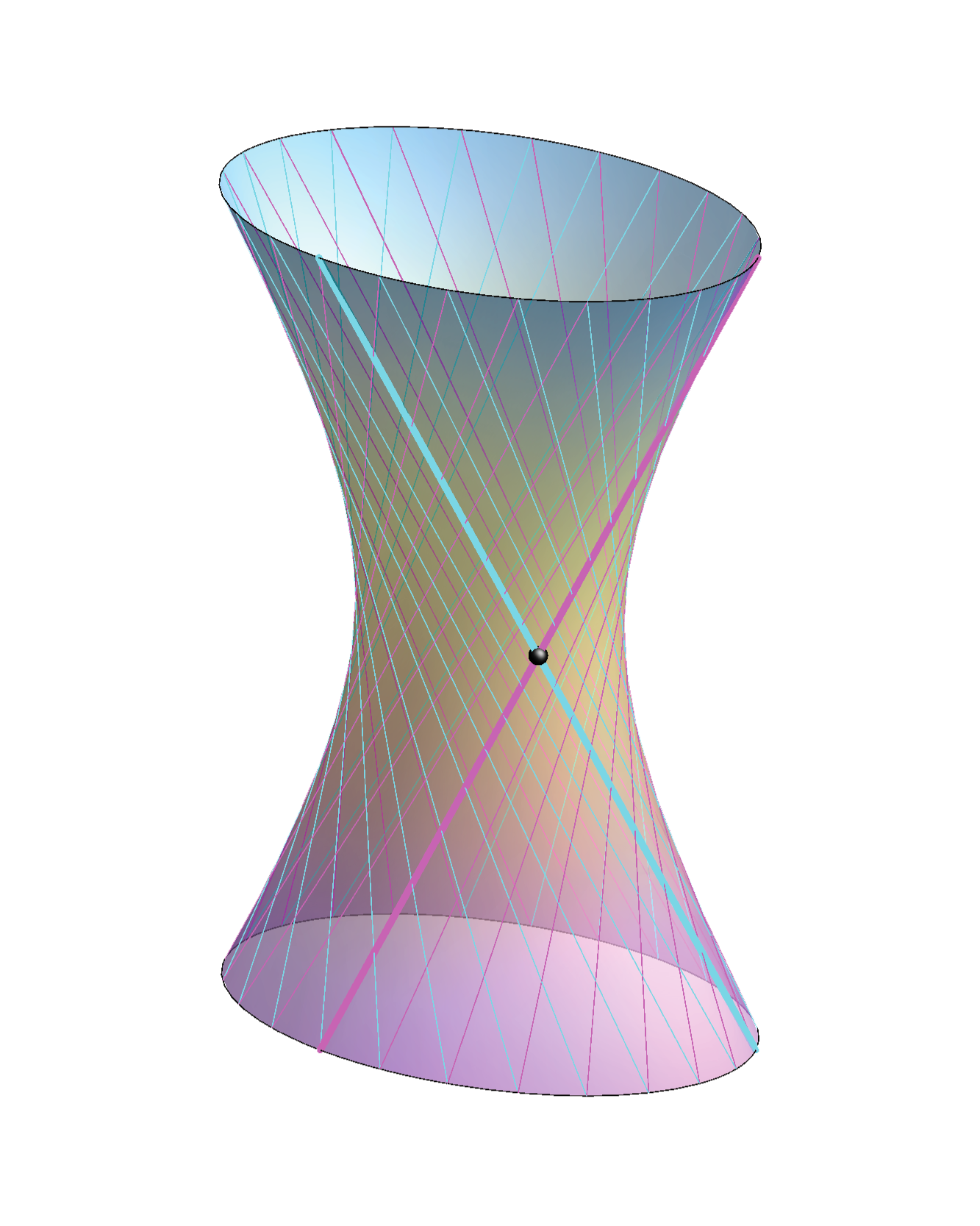one-sheeted hyperboloid of revolution $$\frac{x^2}{a^2}+\frac{y^2}{a^2}-\frac{z^2}{c^2}=1$$ A special case of a one-sheeted elliptic hyperboloid  (for $$a=b$$). It is obtained by rotating a hyperbola about its conjugate axis or by rotating one line about another line when these lines are skew. The rotated line is a ruling belonging to both systems of rulings of this ruled surface.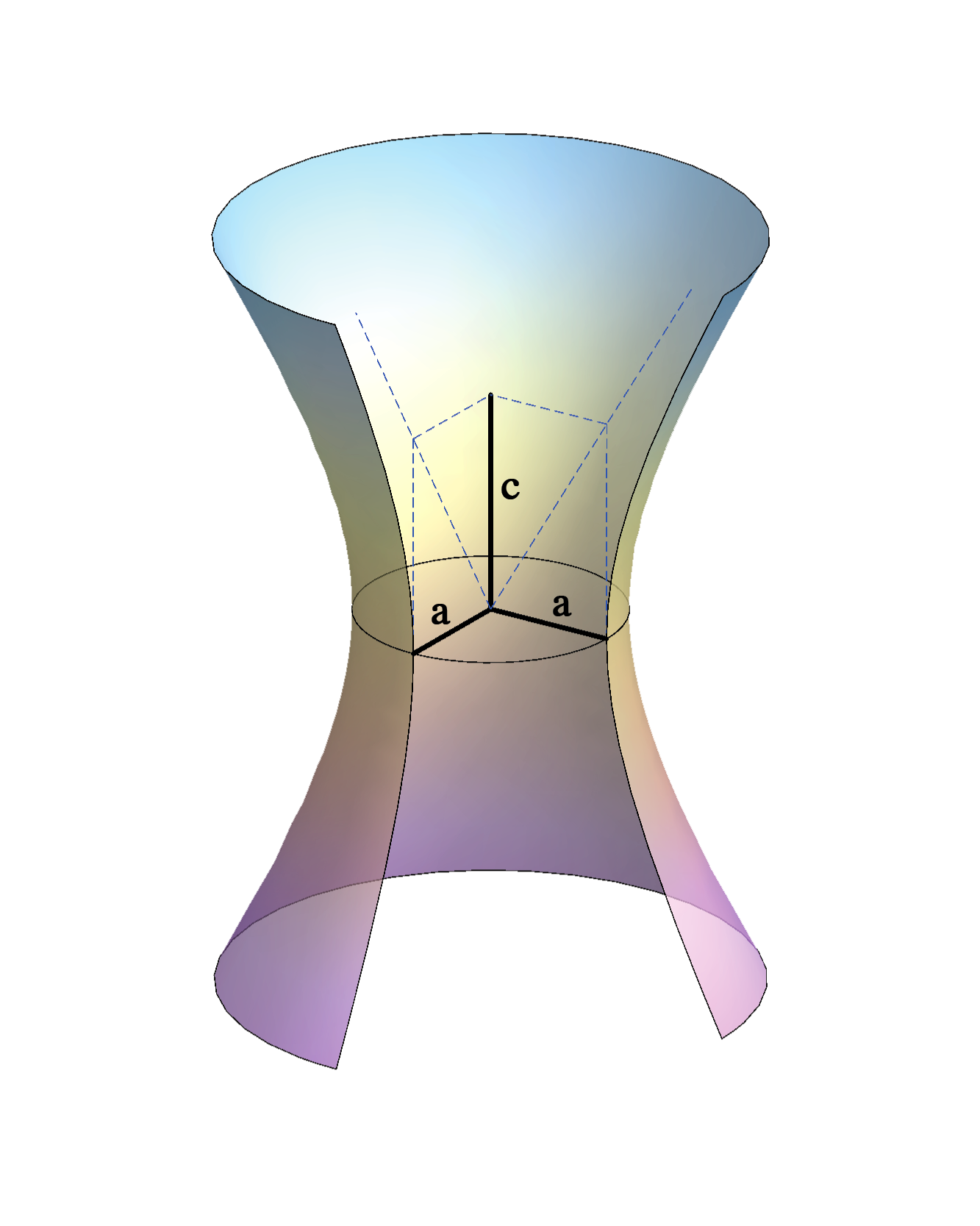right-click $$\rightarrow$$ PLAY right-click $$\rightarrow$$ PLAY more about one-sheeted hyperboloid t LINK

Created by Sonja Gorjanc, translated by Helena Halas and Iva Kodrnja - 3DGeomTeh - Developing project of the University of Zagreb